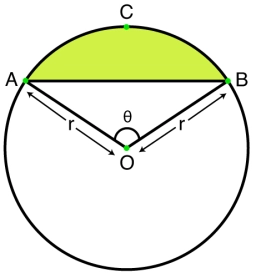# Circumference 81778

Points A and B lie on the circle k. The circumference of the circle k is 40 CM, and the length of the circular arc AB is 10 CM. Determine the size of the angle ABS.

α =  90 °

### Step-by-step explanation:Did you find an error or inaccuracy? Feel free to write us. Thank you!

Tips for related online calculators
Need help calculating sum, simplifying, or multiplying fractions? Try our fraction calculator.
Do you want to convert length units?

#### Grade of the word problem:

We encourage you to watch this tutorial video on this math problem: